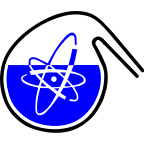#RadChem 2018

13-18 May 2018
Casino Conference Centre
Europe/Prague timezone

## Activated carbon as an effective pertechnetate adsorbent

14 May 2018, 17:15
1h 30m
Gallery (Casino Conference Centre)

### Gallery

#### Casino Conference Centre

Poster Separation Methods, Speciation

### Speaker

Daňo Martin (Czech Technical University in Prague, Faculty of Nuclear Sciences and Physical Engineering, Department of Nuclear Chemistry)

### Description

Activated carbon (AC) is pores space bounded by the carbon atoms. Pores of AC are directive for its application. It was shown that, surface modified form of carbon-based materials, are promising sorbents for separation of various radionuclides. In our study 5 AC adsorbents (A-E) are prepared from cellulose wool by treating with different solutions and carbonized at the temperatures of (700-800) °C for Tc adsorption. They are characterized by FTIR for organic functional groups determination, HRTEM for microscopic imaging, liquid N$_2$ sorption for pore size distribution and specific surface area determination, and acid-base titration conduced potentiometrically for surface charge investigation. Surface area of the sample is within the range of (150-510) m$^{2}$·g$^{-1}$. Samples C and E possess also mesopores. AC samples have a positive surface charge and point of zero charge lies in the range of pH 6-8.
Tc is a fission product with a long half-life $^{97}$Tc (EC; 4.21·10$^{6}$ a), $^{98}$Tc (β$^{-}$; 4.2·10$^{6}$ a), $^{99}$Tc (β$^{-}$; 2.1·10$^{5}$ a) formed in the thermal neutron fission of $^{235}$U and $^{99m}$Tc is the most commonly used diagnostic tool. One option how to remove or control released Tc in the environment, is sorption process. It has been shown that AC has a relatively high efficiency for Tc retaining ($^{99m}$TcO$_4$$^{-}). Adsorption equilibrium of TcO_4$$^{-}$ is reached in few minutes except for sample D (60 minutes). Mechanism of TcO$_4$$^{-} adsorption involves: R-C-OH + TcO_4$$^{-}$ = R-C-OTcO3 + OH$^{-}$ or if phenolic or carboxylic groups are present: R-CO···H + TcO$_4$$^{-} = R-C···H···OTcO_3. Tc can bind direct to the C as -C-OTcO_4 or mechanism involves chemical reduction of the soluble TcO_4$$^{-}$ to TcO$_2$ and its adsorption on the surface. Adsorption of TcO$_4$$^{-} on samples A - E occurs at pH of 2. Presence of anions suppresses adsorption of TcO_4$$^{-}$. HCOO$^{-}$, NO$_3$$^{-}, Br^{-}, Cl^{-}, CH_3COO^{-}, SO_4$$^{2-}$, and ClO$_4$$^{-} are used to investigate the adsorption competition between TcO_4$$^{-}$ and anions. Competition strongly depends on pH, structure of the anions, and standard absolute molar enthalpy of the hydration. The most significant decrease of TcO$_4$$^{-} adsorption is observed in presence of NO_3$$^{-}$ and ClO$_4$$^{-}. It is due to close values of the highest standard absolute molar enthalpies of hydration. Moreover, ClO_4$$^{-}$ has similar tetrahedral structure to TcO$_4$$^{-}. Following order for sample A shows the suppression of TcO_4$$^{-}$ adsorption from the weakest to the strongest (kJ mol$^{-}$): SO$_4$$^{2-} (-1,099) < HCOO^{-} (-384) < CH_3COO^{-} (-374) < Cl^{-} (-359) < Br^{-} (-328) < NO_3$$^{-}$ (-316) < ClO$_4$$^{-} (-205). In order to investigate desorption of TcO_4$$^{-}$ and thus reusability of AC, simple column desorption experiments are carried out. Desorption solutions consist of anions as in the previous paragraph, respectively, at pH 12. Continuous gamma measurements show that 1 M ClO$_4$$^{-} has the "strongest" ability to remove TcO_4$$^{-}$ from AC. Adsorptions of TcO$_4$$^{-} on samples with oxidized and reduced surface are also studied. Immediate adsorption of TcO_4$$^{-}$ makes reduced surface sample preferable to oxidized one. The results obtained in this study improve that activated carbon is an effective sorbent for the separation of the pertechnetate. This work was supported by VEGA Project No. 1/0507/17.

### Primary authors

Daňo Martin (Czech Technical University in Prague, Faculty of Nuclear Sciences and Physical Engineering, Department of Nuclear Chemistry) Galamboš Michal (Comenius University in Bratislava, Faculty of Natural Sciences, Department of Inorganic Chemistry) Viglašová Eva (Comenius University in Bratislava, Faculty of Natural Sciences, Department of Inorganic Chemistry) Rajec Pavol (Comenius University in Bratislava, Faculty of Natural Sciences, Department of Inorganic Chemistry)

### Presentation Materials

There are no materials yet.